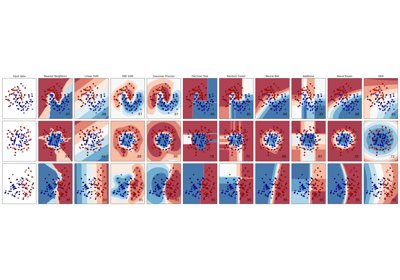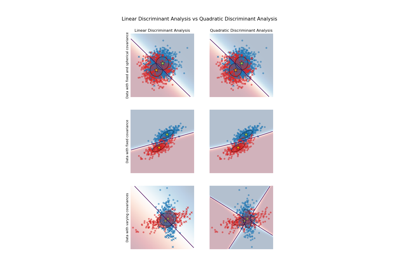# sklearn.discriminant_analysis.QuadraticDiscriminantAnalysis¶

class sklearn.discriminant_analysis.QuadraticDiscriminantAnalysis(*, priors=None, reg_param=0.0, store_covariance=False, tol=0.0001)[source]

A classifier with a quadratic decision boundary, generated by fitting class conditional densities to the data and using Bayes’ rule.

The model fits a Gaussian density to each class.

Read more in the User Guide.

Parameters
priorsndarray of shape (n_classes,), default=None

Class priors. By default, the class proportions are inferred from the training data.

reg_paramfloat, default=0.0

Regularizes the per-class covariance estimates by transforming S2 as S2 = (1 - reg_param) * S2 + reg_param * np.eye(n_features), where S2 corresponds to the scaling_ attribute of a given class.

store_covariancebool, default=False

If True, the class covariance matrices are explicitely computed and stored in the self.covariance_ attribute.

New in version 0.17.

tolfloat, default=1.0e-4

Absolute threshold for a singular value to be considered significant, used to estimate the rank of Xk where Xk is the centered matrix of samples in class k. This parameter does not affect the predictions. It only controls a warning that is raised when features are considered to be colinear.

New in version 0.17.

Attributes
covariance_list of len n_classes of ndarray of shape (n_features, n_features)

For each class, gives the covariance matrix estimated using the samples of that class. The estimations are unbiased. Only present if store_covariance is True.

means_array-like of shape (n_classes, n_features)

Class-wise means.

priors_array-like of shape (n_classes,)

Class priors (sum to 1).

rotations_list of len n_classes of ndarray of shape (n_features, n_k)

For each class k an array of shape (n_features, n_k), where n_k = min(n_features, number of elements in class k) It is the rotation of the Gaussian distribution, i.e. its principal axis. It corresponds to V, the matrix of eigenvectors coming from the SVD of Xk = U S Vt where Xk is the centered matrix of samples from class k.

scalings_list of len n_classes of ndarray of shape (n_k,)

For each class, contains the scaling of the Gaussian distributions along its principal axes, i.e. the variance in the rotated coordinate system. It corresponds to S^2 / (n_samples - 1), where S is the diagonal matrix of singular values from the SVD of Xk, where Xk is the centered matrix of samples from class k.

classes_ndarray of shape (n_classes,)

Unique class labels.

Examples

>>> from sklearn.discriminant_analysis import QuadraticDiscriminantAnalysis
>>> import numpy as np
>>> X = np.array([[-1, -1], [-2, -1], [-3, -2], [1, 1], [2, 1], [3, 2]])
>>> y = np.array([1, 1, 1, 2, 2, 2])
>>> clf.fit(X, y)
>>> print(clf.predict([[-0.8, -1]]))



Methods

 Apply decision function to an array of samples. fit(X, y) Fit the model according to the given training data and parameters. get_params([deep]) Get parameters for this estimator. Perform classification on an array of test vectors X. Return log of posterior probabilities of classification. Return posterior probabilities of classification. score(X, y[, sample_weight]) Return the mean accuracy on the given test data and labels. set_params(**params) Set the parameters of this estimator.
decision_function(X)[source]

Apply decision function to an array of samples.

The decision function is equal (up to a constant factor) to the log-posterior of the model, i.e. log p(y = k | x). In a binary classification setting this instead corresponds to the difference log p(y = 1 | x) - log p(y = 0 | x). See Mathematical formulation of the LDA and QDA classifiers.

Parameters
Xarray-like of shape (n_samples, n_features)

Array of samples (test vectors).

Returns
Cndarray of shape (n_samples,) or (n_samples, n_classes)

Decision function values related to each class, per sample. In the two-class case, the shape is (n_samples,), giving the log likelihood ratio of the positive class.

fit(X, y)[source]

Fit the model according to the given training data and parameters.

Changed in version 0.19: store_covariances has been moved to main constructor as store_covariance

Changed in version 0.19: tol has been moved to main constructor.

Parameters
Xarray-like of shape (n_samples, n_features)

Training vector, where n_samples is the number of samples and n_features is the number of features.

yarray-like of shape (n_samples,)

Target values (integers)

get_params(deep=True)[source]

Get parameters for this estimator.

Parameters
deepbool, default=True

If True, will return the parameters for this estimator and contained subobjects that are estimators.

Returns
paramsmapping of string to any

Parameter names mapped to their values.

predict(X)[source]

Perform classification on an array of test vectors X.

The predicted class C for each sample in X is returned.

Parameters
Xarray-like of shape (n_samples, n_features)
Returns
Cndarray of shape (n_samples,)
predict_log_proba(X)[source]

Return log of posterior probabilities of classification.

Parameters
Xarray-like of shape (n_samples, n_features)

Array of samples/test vectors.

Returns
Cndarray of shape (n_samples, n_classes)

Posterior log-probabilities of classification per class.

predict_proba(X)[source]

Return posterior probabilities of classification.

Parameters
Xarray-like of shape (n_samples, n_features)

Array of samples/test vectors.

Returns
Cndarray of shape (n_samples, n_classes)

Posterior probabilities of classification per class.

score(X, y, sample_weight=None)[source]

Return the mean accuracy on the given test data and labels.

In multi-label classification, this is the subset accuracy which is a harsh metric since you require for each sample that each label set be correctly predicted.

Parameters
Xarray-like of shape (n_samples, n_features)

Test samples.

yarray-like of shape (n_samples,) or (n_samples, n_outputs)

True labels for X.

sample_weightarray-like of shape (n_samples,), default=None

Sample weights.

Returns
scorefloat

Mean accuracy of self.predict(X) wrt. y.

set_params(**params)[source]

Set the parameters of this estimator.

The method works on simple estimators as well as on nested objects (such as pipelines). The latter have parameters of the form <component>__<parameter> so that it’s possible to update each component of a nested object.

Parameters
**paramsdict

Estimator parameters.

Returns
selfobject

Estimator instance.

## Examples using sklearn.discriminant_analysis.QuadraticDiscriminantAnalysis¶